# Samacheer Kalvi Books: Tamilnadu State Board Text Books Solutions

## Samacheer Kalvi 7th Maths Direct and Inverse Proportion Notes PDF Download: Tamil Nadu STD 7th Maths Direct and Inverse Proportion NotesSamacheer Kalvi 7th Maths Direct and Inverse Proportion Notes PDF Download: Tamil Nadu STD 7th Maths Direct and Inverse Proportion Notes

## Samacheer Kalvi 7th Maths Direct and Inverse Proportion Notes PDF Download

We bring to you specially curated Samacheer Kalvi 7th Maths Direct and Inverse Proportion Notes PDF which have been prepared by our subject experts after carefully following the trend of the exam in the last few years. The notes will not only serve for revision purposes, but also will have several cuts and easy methods to go about a difficult problem.

 Board Tamilnadu Board Study Material Notes Class Samacheer Kalvi 7th Maths Subject 7th Maths Chapter Direct and Inverse Proportion Format PDF Provider Samacheer Kalvi Books

## How to Download Samacheer Kalvi 7th Maths Direct and Inverse Proportion Notes PDFs?

2. Click on the Samacheer Kalvi 7th Maths Notes PDF.
3. Look for your preferred subject.
4. Now download the Samacheer Kalvi 7th Maths Direct and Inverse Proportion notes PDF.

## Tamilnadu Samacheer Kalvi 7th Maths Solutions Term 1 Chapter 4 Direct and Inverse Proportion Intext Questions

Try These (Text Book Page No. 72)

Question 1.
Find the ratio of the number of circles to number of squares.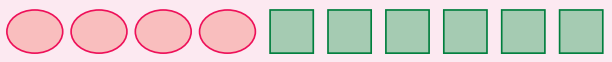Solution:
Number of circles = 4 ;
Number of squares = 6
Number of circles : Number of squares = 4 : 6 = 2 : 3

Question 2.
Find the ratio (i) 555 g to 5 kg
(ii) 21 km to 175 m.
Solution:
(i) 1 kg = 1000 g
∴ 5 kg = 5000 g
∴ 555 g : 5 kg = 555 g : 5000 g = 111 : 1000

(ii) 21 km to 175m
1 km = 1000 m
21 km = 21 × 1000 = 21,000m
∴ 21 km : 175 m = 21000 : 175 = 120 : 1

Question 3.
Find the value of x in the following proportions :
(i) 110 8: 88
(ii) x : 26 :: 5: 65
Solution:
(i) Given 110 : x :: 8 : 88
Product of the means = Product of the extremes
x × 8 = 110 × 88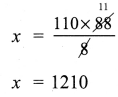(ii) x : 26 :: 5 : 65
Product of the means = Product of the extremes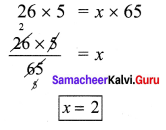Try this (Text Book Page No. 74)

Question 1.
The number of chocolates to be distributed to the number of children. Is this statement in direct proportion?
Solution:
Let the number of students be x and the number of chocolates be y. As the valve of x increases also correspondingly increases.
ie xy=k
∴ x and y are in direct proportion.

Try This (Text book Page No. 74)

Question 2.
Observe the following 5 squares of different sides given in the graph sheet.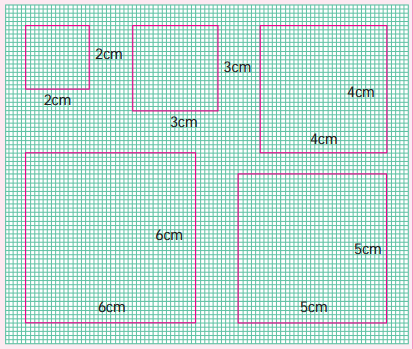The measures of the sides are recorded in the table given below. Find the corresponding perimeter and the ratios of each of these with the sides given and complete the table.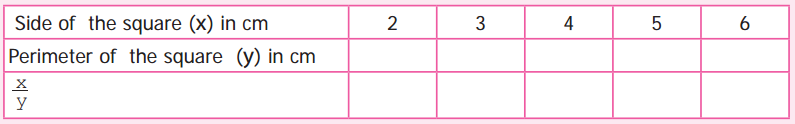From the information so obtained state whether the side of a square is in direct proportion to the perimeter of the square.
Solution:
Perimeter of the square y = 4x, units where x is the side of a square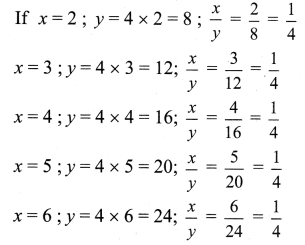Completing the table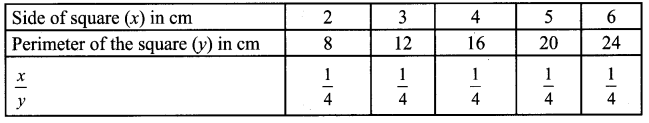From the above table we find that as x increases y also increased in such a way that xy=14, constant.
∴ Side of a square is in direct proportion to the perimeter of the square.

Try this (Text book Page No. 75)

Question 3.
When a fixed amount is deposited for a fixed rate of interest, the simple interest changes proportionally with the number of years it is being deposited. Can you find any other examples of such kind.
Solution:
Some other examples of such kind are
(i) Cost of book and number of books
(ii) Distance and time to travel
(iii) Men workers and wages.

Exercise 4.2

Try this (Text book Page No. 78)

Question 1.
Think of an example in real life where two variable are inversely proportional.
Solution:
Example of inverse proportion are
(i) Men working and the amount of work.
(ii) Speed and time to travel

Try These (Text book Page No. 78)

Question 1.
Complete the table given below and find the type of proportion.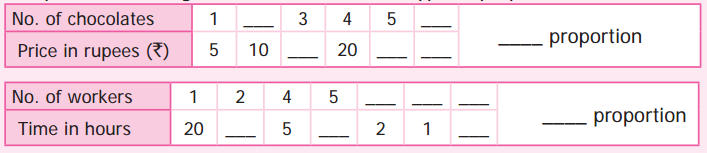Solution: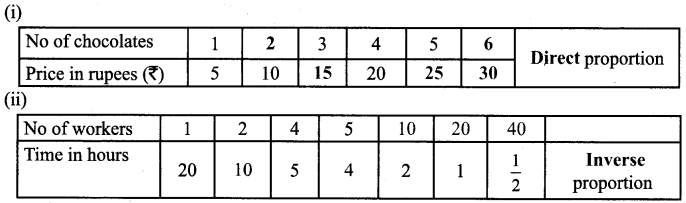Question 2.
Read the following examples and group them in two categories.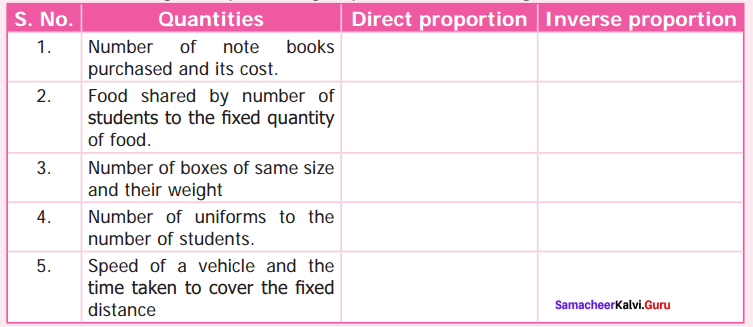Solution: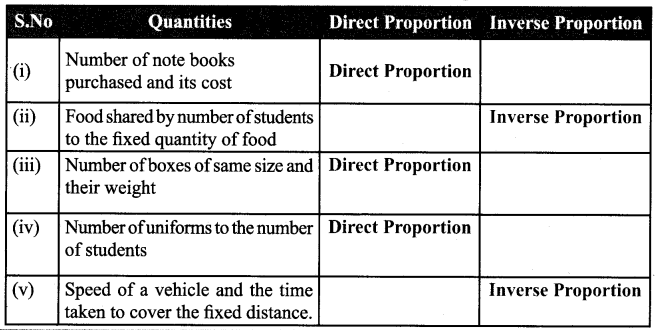## How to Prepare using Samacheer Kalvi 7th Maths Direct and Inverse Proportion Notes PDF?

Students must prepare for the upcoming exams from Samacheer Kalvi 7th Maths Direct and Inverse Proportion Notes PDF by following certain essential steps which are provided below.

• Use Samacheer Kalvi 7th Maths Direct and Inverse Proportion notes by paying attention to facts and ideas.
• Pay attention to the important topics
• Refer TN Board books as well as the books recommended.
• Correctly follow the notes to reduce the number of questions being answered in the exam incorrectly
• Highlight and explain the concepts in details.

## Frequently Asked Questions on Samacheer Kalvi 7th Maths Direct and Inverse Proportion Notes

#### How to use Samacheer Kalvi 7th Maths Direct and Inverse Proportion Notes for preparation??

Read TN Board thoroughly, make separate notes for points you forget, formulae, reactions, diagrams. Highlight important points in the book itself and make use of the space provided in the margin to jot down other important points on the same topic from different sources.

#### How to make notes for Samacheer Kalvi 7th Maths Direct and Inverse Proportion exam?

Read from hand-made notes prepared after understanding concepts, refrain from replicating from the textbook. Use highlighters for important points. Revise from these notes regularly and formulate your own tricks, shortcuts and mnemonics, mappings etc.
Share: